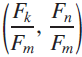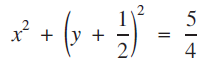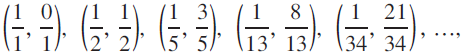Hostname: page-component-5db6c4db9b-k8dct Total loading time: 0 Render date: 2023-03-23T15:02:29.063Z Has data issue: true Feature Flags: { "useRatesEcommerce": false } hasContentIssue true

# Fibonacci Fraction Circles

Published online by Cambridge University Press:  24 February 2022

## Extract

We define a Fibonacci fraction circle to be a circle passing through an infinite number of points whose coordinates are of the form$\left( {{{{F_k}} \over {{F_m}}},{{{F_n}} \over {{F_m}}}} \right)$ , where the F’s are Fibonacci numbers (0, 1, 1, 2, 3, 5, 8, 13, 21, 34, …). For instance, the circle (1)$${x^2} + {\left( {y + {1 \over 2}} \right)^2} = {5 \over 4}$$
passes through the points$\left( {{1 \over 1},{0 \over 1}} \right),\,\left( {{1 \over 2},{1 \over 2}} \right),\,\left( {{1 \over 5},{3 \over 5}} \right),\,\left( {{1 \over {13}},{8 \over {13}}} \right),\left( {{1 \over {34}},{{21} \over {34}}} \right),\,...,$ which can be easily checked. See Figure 1. The purpose of this paper is to find other Fibonacci fraction circles. Several of these circles have been discovered independently by Kocik. 

Type
Articles
Information
The Mathematical Gazette , March 2022 , pp. 1 - 8